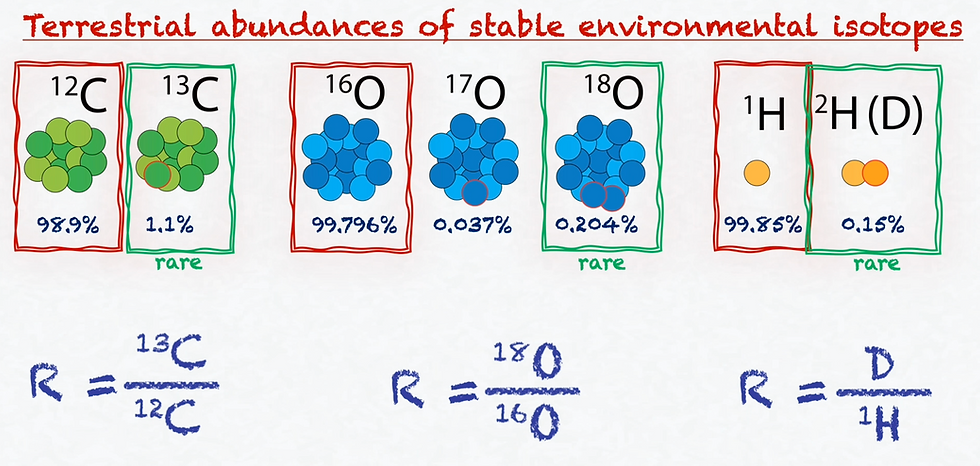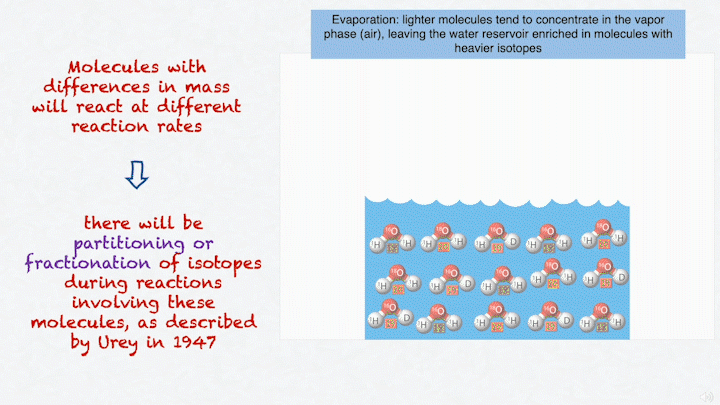top of page
Buscar

# Stable isotopes and the delta notation

Stable isotopes serve as tracers of many processes, providing information on how chemical reactions proceed in nature. To quantify these tracers, we use the delta notation.

To understand the meaning of the delta notation, let's use as examples hydrogen, carbon and oxygen.Carbon has two isotopes of interest, carbon 13 (the heavy one) with an abundance of 1.1%, and carbon 12 (the lighter one) with the abundance of 98.9%. Likewise, oxygen has three isotopes of interest, the heavy oxygen 18, with an abundance of 0.204%, the intermediate oxygen 17, with an abundance of 0.037%, and the lighter oxygen 16, with an abundance of 99.796%. Hydrogen has two stable isotopes. Hydrogen two, called deuterium, has an abundance of 0.15%, and hydrogen one, just called hydrogen, has an abundance of 99.85%.

Stable environmental isotopes are measured as the ratio between the two most abundant isotopes of even element.You may have noticed that the most abundant one is always the lighter one and the second most abundant is always the heavy one with a very low abundance, and this is why we call it rare isotope. We are always looking at the difference in abundance between the rare (the heavier) and the most abundant (lighter) isotope.

Now, each of these heavy and light isotopes may be part of molecules that will have different masses according to the isotopes they are composed of. For example, some of the arrangements of isotopes in water produces compounds with different masses of 20, 19 and 18:Molecules with differences in mass have a different reaction rates. This means that there will be partitioning or fractionation of isotopes during reactions involving these molecules, as described by Urey in 1947. Fractionation of isotopes will modify these ratios slightly for any given compound containing the stabilizer. And, by slightly, I mean that variations are seeing only it’s the fifth or sixth decimal place.Measuring an absolute isotopic ratio or abundance is not easily done. Luckily what we are interested in doing is comparing the variation in the concentration of a stable isotope to a known concentration, rather than measuring a true ratio for the sample, which is difficult to do. We do this by measuring an apparent ratio in the sample, which can be easily done by gas source mass spectrometry, and then measuring a known reference on the same machine at the same time. This way, we can compare our sample to the reference.This is expressed using the delta notation. The delta of the nuclide is the isotopic concentration expressed as the difference between the measured ratios of the sample and the reference over the measured ratio of the reference. These ratios, represented by the letter R, are the same ratios we mentioned before for oxygen 18 and oxygen 16, deuterium and hydrogen, and carbon 13 and carbon 12.

Because fractionation processes will impart very little variations in isotope concentrations, delta values are expressed as parts per thousand or permil difference from the reference:We reorganize the expression for it to become its more known form:Note that the nuclide here will always be the heavy isotope, like oxygen 18. The reference will always have to be stated, and will depend on the type of sample we are working with. Let’s say we are talking about 𝛿18O in a water sample. The standard reference for water according to the United Nations international atomic energy agency (IAEA), is VSMOW, which stands for Vienna standard mean ocean water.Using the expression, we determine the delta value of VSMOW.The delta values in our samples will probably have a different value than that of VSMOW. If we have a delta value permil that is positive, let’s say +10 permil, this means that our sample has 10 permil or 1% more oxygen 18 than VSMOW. In this case, we usually say that the sample is ENRICHED by 10 permil. If we have a delta value that is negative, let’s say -5 per mill, this means that our sample has five permil or 0.5% less oxygen 18 then VSMOW.Having one sample that is 10 permil enriched and another that is five permil depleted from VSMOW, which is the standard value for mean ocean water, can indicate that the samples went through different processes in the hydrological cycle in the past. For instance, let's say we were dealing with water samples. Waters from highly evaporative lakes tend to have very enriched 𝛿18O values whereas waters derived from glacier melting tend to have very depleted 𝛿18O values.

Just like there is VSMOW for hydrogen and oxygen in H2O, there are other accepted standards for other materials and isotopes of interest, as shown in this table:For instance, VPDB is the standard used for both carbon and oxygen in carbonates.I hope this summary was helpful for you!

575 visualizações1 comentário
bottom of page